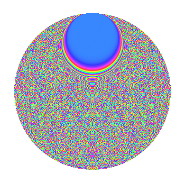# Properties

 Label 3009.2.biLevel 3009 Weight 2 Character orbit bi Rep. character $$\chi_{3009}(19,\cdot)$$ Character field $$\Q(\zeta_{232})$$ Dimension 20160 Sturm bound 720

# Related objects

## Defining parameters

 Level: $$N$$ = $$3009 = 3 \cdot 17 \cdot 59$$ Weight: $$k$$ = $$2$$ Character orbit: $$[\chi]$$ = 3009.bi (of order $$232$$ and degree $$112$$) Character conductor: $$\operatorname{cond}(\chi)$$ = $$1003$$ Character field: $$\Q(\zeta_{232})$$ Sturm bound: $$720$$

## Dimensions

The following table gives the dimensions of various subspaces of $$M_{2}(3009, [\chi])$$.

Total New Old
Modular forms 40768 20160 20608
Cusp forms 39872 20160 19712
Eisenstein series 896 0 896

## Trace form

 $$20160q + O(q^{10})$$ $$20160q + 32q^{14} + 720q^{16} + 16q^{17} - 16q^{19} - 32q^{22} - 16q^{23} - 64q^{26} + 16q^{29} - 32q^{31} + 80q^{32} - 32q^{33} + 32q^{34} - 64q^{35} - 16q^{37} - 32q^{39} + 48q^{40} + 32q^{41} + 32q^{42} + 32q^{43} - 32q^{46} - 864q^{50} + 96q^{52} + 16q^{53} - 80q^{56} + 32q^{57} - 48q^{58} - 16q^{59} - 96q^{61} + 64q^{65} + 32q^{66} - 496q^{67} + 80q^{68} + 64q^{69} + 64q^{70} + 496q^{71} + 32q^{73} - 96q^{74} - 32q^{75} + 128q^{76} - 160q^{77} - 64q^{78} + 16q^{80} + 16q^{82} - 48q^{85} + 128q^{86} - 48q^{87} - 64q^{88} + 32q^{91} + 224q^{92} - 32q^{93} - 16q^{94} - 64q^{95} - 16q^{97} + O(q^{100})$$

## Decomposition of $$S_{2}^{\mathrm{new}}(3009, [\chi])$$ into newform subspaces

The newforms in this space have not yet been added to the LMFDB.

## Decomposition of $$S_{2}^{\mathrm{old}}(3009, [\chi])$$ into lower level spaces

$$S_{2}^{\mathrm{old}}(3009, [\chi]) \cong$$ $$S_{2}^{\mathrm{new}}(1003, [\chi])$$$$^{\oplus 2}$$

## Hecke Characteristic Polynomials

There are no characteristic polynomials of Hecke operators in the database